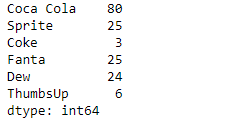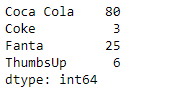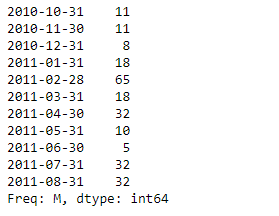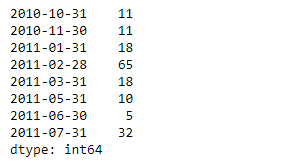# Python | Pandas Series.drop()

• Last Updated : 15 Feb, 2019

Pandas series is a One-dimensional ndarray with axis labels. The labels need not be unique but must be a hashable type. The object supports both integer- and label-based indexing and provides a host of methods for performing operations involving the index.

Pandas` Series.drop()` function return Series with specified index labels removed. It remove elements of a Series based on specifying the index labels.

Syntax: Series.drop(labels=None, axis=0, index=None, columns=None, level=None, inplace=False, errors=’raise’)

Parameter :
labels : Index labels to drop.
axis : Redundant for application on Series.
index, columns : Redundant for application on Series, but index can be used instead of labels.
level : For MultiIndex, level for which the labels will be removed.
inplace : If True, do operation inplace and return None.
errors : If ‘ignore’, suppress error and only existing labels are dropped.

Returns : dropped : pandas.Series

Example #1: Use `Series.drop()` function to drop the values corresponding to the passed index labels in the given series object.

 `# importing pandas as pd``import` `pandas as pd`` ` `# Creating the Series``sr ``=` `pd.Series([``80``, ``25``, ``3``, ``25``, ``24``, ``6``])`` ` `# Create the Index``index_ ``=` `[``'Coca Cola'``, ``'Sprite'``, ``'Coke'``, ``'Fanta'``, ``'Dew'``, ``'ThumbsUp'``]`` ` `# set the index``sr.index ``=` `index_`` ` `# Print the series``print``(sr)`

Output :Now we will use `Series.drop()` function to drop the values corresponding to the passed index labels in the given series object.

 `# drop the passed labels``result ``=` `sr.drop(labels ``=` `[``'Sprite'``, ``'Dew'``]) `` ` `# Print the result``print``(result)`

Output :As we can see in the output, the `Series.drop()` function has successfully dropped the entries corresponding to the passed index labels.

Example #2 : Use `Series.drop()` function to drop the values corresponding to the passed index labels in the given series object.

 `# importing pandas as pd``import` `pandas as pd`` ` `# Creating the Series``sr ``=` `pd.Series([``11``, ``11``, ``8``, ``18``, ``65``, ``18``, ``32``, ``10``, ``5``, ``32``, ``32``])`` ` `# Create the Index``index_ ``=` `pd.date_range(``'2010-10-09'``, periods ``=` `11``, freq ``=``'M'``)`` ` `# set the index``sr.index ``=` `index_`` ` `# Print the series``print``(sr)`

Output :Now we will use `Series.drop()` function to drop the values corresponding to the passed index labels in the given series object.

 `# drop the passed labels``result ``=` `sr.drop(labels ``=` `[pd.Timestamp(``'2010-12-31'``),``                           ``pd.Timestamp(``'2011-04-30'``), pd.Timestamp(``'2011-08-31'``)])`` ` `# Print the result``print``(result)`

Output :As we can see in the output, the `Series.drop()` function has successfully dropped the entries corresponding to the passed index labels.

My Personal Notes arrow_drop_up